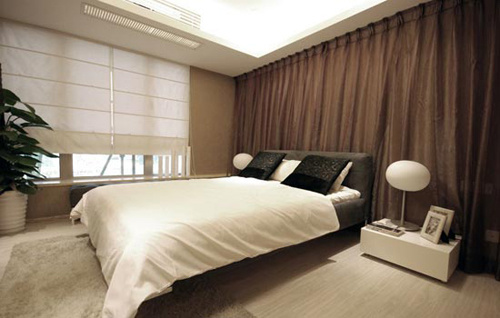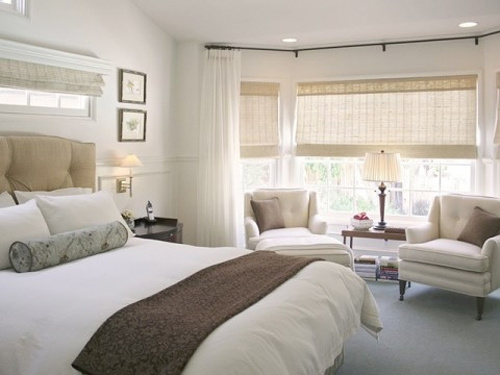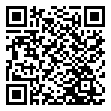|

# 破财损健康的风水禁忌 碰见了快躲开!

人们常问，到底是金钱重要还是健康重要。其实想要拥有一个舒适安定的生活，这两样都不可缺少。家居风水是一门高深的学问，这背后隐含着非常多的讲究，需要我们在日常家居布置中多加留意，才不会让自己的健康和财运受到损害。下面，就跟着小编一起来了解一下相关的知识吧。1、户型呈下行阶梯浊水形。有些家居由于采光的原因将房屋设计成阶梯状，远望其形如锯齿形或水波纹，故家居风水学中把左后方和右后方呈阶梯状的房屋叫下行阶梯浊水形。其风水暗示是宅主在财运、事业等方面将会是一路走下坡路之象，尤其是财运方面，因为水主财也。不过，浊水者说明财来路不正，去向也会不明。

2、房型忌过于狭长浮木形。一般来讲，过于狭长的房屋是指房屋的长度超过宽度四倍以上者，此犯家居风水中的浮木煞。在风水学中，长为阳，宽为阴，此明显为长宽比例失调，造成阴阳失衡。过于狭长浮木形的住宅其不利的风水后果是事业上的冒进、根基不实等因素造成功亏一篑、钱财耗尽而漂泊异乡。

3、入门先见厨厕，退运之宅。所有的屋子，入门必见客厅。现代的建筑设计，有时为了考虑空间的配置，一进门往往先见到厨房、餐厅或浴厕。这是阳宅的大忌，也不合常理，居住其中，家运必衰。

4、前大后小棺材形。房屋前面大后面小、前面宽后面窄，形如棺材，故风水学中称之为棺材煞。棺材形的房屋极不聚气，房屋后面小说明其容量有限，前面大说明其耗泄量却是无限。因此，前大后小棺材形的房屋其不利的风水后果是易产生事业上虎头蛇尾、有志难伸、收入越来越少而破耗越来越多等。

5、拐弯抹角轭头形。此即为房屋的形状如“7”字形或者“L”形之类。家居风水学上称此类型房屋犯轭头煞。此户型的房屋主要是房屋明显由两个部分组成，并且房屋的太极点往往不在房屋之内，故此类房屋非常不聚气。其不利的风水后果是易因夫妻对立、事业波折、小人不断、破财连连。

6、大门直通到底，麻烦不断居家忌象宾馆饭店一样一条长廊连着一排数个房间，否则易发生外遇及私奔现象，难得平安。

7、屋内房门，开门方向应一致。屋内房门，开门方向应一致，这一点从门把手就可以断定。最忌一扇左开，一扇右开。

8、浴厕改成卧房。现代大楼管线整体施工，所以整栋大楼浴厕都设在同一地方。如果将浴厕改为卧房，势必造成睡在楼上和楼下两层的浴厕当中，而浴厕本为潮湿、不洁之所，夹在当中必然对环境卫生有所影响，另外当楼上马桶、水管一开动也绝对会影响到您的安宁，所以对人的身心健康造成伤害。

9、卧室面积超过20平方米。古代风水理论指出“屋大人少，是凶屋”，认为“大房子会吸人气”。因此，即使是皇帝的寝宫，面积也不会超过20平方米。 其实风水中所说的“人气”就是我们后来发现的“人体能量场”。人体是一个能量体，无时无刻不在向外散发能量，就像工作中的空调，房屋面积越大所耗损的能量就越多。因此，卧室面积过大肯恶导致人体因耗能过多而免疫力下降、无精打采、判断力下降、做出错误决定、甚至“倒霉”生病。 专家建议：卧室面积控制在10-20平方米为佳。10、卧房形状为斜边或是多角形状。 斜边容易造成视线上的错觉，多角容易造成压迫，因而增加人的精神负担，长期下来容易罗患疾病及发生意外。

11、卧房昏暗。 卧房应设有窗户，除了空气得以流通，白天更可以采光，使人精神畅快，而晚间窗户应备有窗帘，挡住户外夜光，使人容易入眠。

12、浴厕设在走廊尽头，大凶。屋内如有走廊，浴厕只宜设在走廊边上，不可设在尽头，否则大凶。

13、房子建在三角地的尖头，如一面旗子一样摇摆不定。导致宅主事业不稳，做什么都没有常性，三分钟的热度过了就玩完，终究啥事也办不成。如果以前有积蓄，会坐吃山空，此风水易出偷盗为生之人。

14、房门正对卫生间。卫生间是供人排泄的地方，容易产生秽气和湿气，所以正对房门会对卧房的空气产生影响，对人的生体健康有害。

15、门正对厨房或和厨房相邻。厨房炉火煎炒、排出油烟，容易影响正对的房门，危害人体健康，并且使工作表现不稳定。厨房是生火之处，甚为燥热，所以也不宜与卧房相邻，尤其是睡床紧贴炉灶的墙。

16、柱角冲射不利婚。不论男性或女性之个人房间内，皆要避免柱角冲射，否则必会影响情绪和健康，对恋爱及婚姻亦不利，务必及早补救。

17、隔角煞冲射，宜盆栽抵挡。要想居家平安健康，在可能的情况下，应选择四周没有屋角射来成隔角煞的房屋。

18、房门对镜子。镜子有反射作用，在风水上可将煞气反射回去，所以可挡凶煞。但是镜子对着房门会将凶煞冲克照进卧房，招来不好运势。

19、镜子与落地门窗对床。镜子是用来挡煞，作用是把煞气反射回去，所以不可对床。尤其人们从睡梦中醒来，在意识不很清楚时，容易被映在镜子或落地窗里的自己所惊吓。

20、睡床或床头对正房门。 睡觉时最讲求安全、安静和稳定，房门是进出房间必经之所，因此房门不可对正睡床或床头。否则睡床上的人容易缺乏安全感，并且有损健康。21、床头紧贴窗口。窗户为理气进出之所，所以床头贴近窗口容易犯冲。在睡床上的人因看不见头上的窗口，容易缺乏安全感，造成精神紧张，影响健康。

22、床头在横梁下。天花板宜平坦，忌有横梁。横梁在心理上容易产生重体的感觉，尤其人睡在横梁之下会感受到莫大的压力，造成精神上的压迫，影响健康、事业。

23、床头不靠墙壁。人平躺时不容易看见头顶上，所以床头宜靠墙、避免露空，而减少安全感。否则睡在床上的人，容易精神恍惚、疑神疑鬼，影响健康、事业。

24、床紧贴地面。床面应离开地面50公分左右，床底必需保持清洁，不宜堆积杂物。离开地面，并且不堆积杂物能保持床底空气畅通，减少地面湿气渗透入床垫，而影响健康。

25、卧房摆过多的植物。过多的花草植物容易聚集阴气，并且植物于晚间吸收氧气、释放二氧化碳，所以容易影响人的身体健康。

26、电器过多，尤其电视正对床脚。卧室内电器过多在风水上被称为“火宅”，影响健康。现代医学理论也指出，电器辐射确实损害人体健康。脚是人的第二心脏，处于待机状态的电视若正对床脚，其辐射更容易影响双脚的经络运行及血液循环。 专家建议：少在卧室摆放电器，尤其不要将电视正对床脚，不使用时拔掉电源。

27、窗口大，朝东或朝西。风水师指出睡在窗口大、朝东或朝西的房间中容易因“光煞”导致“血光之灾”。因为在朝东或朝西的房间，早上或下午猛烈的阳光会导致卧室内光线过强，刺激神经影响休息，导致失眠，更使人变得不冷静、冲动易怒。

28、床正上方的屋顶装有吊灯。风水上将“床正上方的屋顶装有吊灯”称为“吊灯压床”，认为“煞气重”。对健康不利。现代心理学研究发现，床正上方的屋顶若装有吊灯，确实会给人以心理暗示，增加人心理压力，影响内分泌，进而引起失眠、噩梦、呼吸系统急病等一系列健康问题。 专家建议：保持床正上方屋顶的空旷，在床边使用光线柔和的落地灯或台灯。

29、客厅在屋子正中大吉。一般住宅，如果起居室或客厅设在整幢房子的正中间，这是一种大吉之象，可使家运昌隆。

30、横梁压顶，影响情绪与健康。横梁最忌压在床头、书桌及餐桌上方，如实在无法避免，也要设计天花板，将之挡住，否则就会影响居者的情绪与健康，事业运亦会受阻。

`声明：本文由入驻焦点开放平台的作者撰写，除焦点官方账号外，观点仅代表作者本人，不代表焦点立场错误信息举报电话： 400-099-0099，邮箱：jubao@vip.sohu.com，或点此进行意见反馈，或点此进行举报投诉。`A B C D E F G H J K L M N P Q R S T W X Y Z
A - B - C - D - E
• A
• 鞍山
• 安庆
• 安阳
• 安顺
• 安康
• 澳门
• B
• 北京
• 保定
• 包头
• 巴彦淖尔
• 本溪
• 蚌埠
• 亳州
• 滨州
• 北海
• 百色
• 巴中
• 毕节
• 保山
• 宝鸡
• 白银
• 巴州
• C
• 承德
• 沧州
• 长治
• 赤峰
• 朝阳
• 长春
• 常州
• 滁州
• 池州
• 长沙
• 常德
• 郴州
• 潮州
• 崇左
• 重庆
• 成都
• 楚雄
• 昌都
• 慈溪
• 常熟
• D
• 大同
• 大连
• 丹东
• 大庆
• 东营
• 德州
• 东莞
• 德阳
• 达州
• 大理
• 德宏
• 定西
• 儋州
• 东平
• E
• 鄂尔多斯
• 鄂州
• 恩施
F - G - H - I - J
• F
• 抚顺
• 阜新
• 阜阳
• 福州
• 抚州
• 佛山
• 防城港
• G
• 赣州
• 广州
• 桂林
• 贵港
• 广元
• 广安
• 贵阳
• 固原
• H
• 邯郸
• 衡水
• 呼和浩特
• 呼伦贝尔
• 葫芦岛
• 哈尔滨
• 黑河
• 淮安
• 杭州
• 湖州
• 合肥
• 淮南
• 淮北
• 黄山
• 菏泽
• 鹤壁
• 黄石
• 黄冈
• 衡阳
• 怀化
• 惠州
• 河源
• 贺州
• 河池
• 海口
• 红河
• 汉中
• 海东
• 怀来
• I
• J
• 晋中
• 锦州
• 吉林
• 鸡西
• 佳木斯
• 嘉兴
• 金华
• 景德镇
• 九江
• 吉安
• 济南
• 济宁
• 焦作
• 荆门
• 荆州
• 江门
• 揭阳
• 金昌
• 酒泉
• 嘉峪关
K - L - M - N - P
• K
• 开封
• 昆明
• 昆山
• L
• 廊坊
• 临汾
• 辽阳
• 连云港
• 丽水
• 六安
• 龙岩
• 莱芜
• 临沂
• 聊城
• 洛阳
• 漯河
• 娄底
• 柳州
• 来宾
• 泸州
• 乐山
• 六盘水
• 丽江
• 临沧
• 拉萨
• 林芝
• 兰州
• 陇南
• M
• 牡丹江
• 马鞍山
• 茂名
• 梅州
• 绵阳
• 眉山
• N
• 南京
• 南通
• 宁波
• 南平
• 宁德
• 南昌
• 南阳
• 南宁
• 内江
• 南充
• P
• 盘锦
• 莆田
• 平顶山
• 濮阳
• 攀枝花
• 普洱
• 平凉
Q - R - S - T - W
• Q
• 秦皇岛
• 齐齐哈尔
• 衢州
• 泉州
• 青岛
• 清远
• 钦州
• 黔南
• 曲靖
• 庆阳
• R
• 日照
• 日喀则
• S
• 石家庄
• 沈阳
• 双鸭山
• 绥化
• 上海
• 苏州
• 宿迁
• 绍兴
• 宿州
• 三明
• 上饶
• 三门峡
• 商丘
• 十堰
• 随州
• 邵阳
• 韶关
• 深圳
• 汕头
• 汕尾
• 三亚
• 三沙
• 遂宁
• 山南
• 商洛
• 石嘴山
• T
• 天津
• 唐山
• 太原
• 通辽
• 铁岭
• 泰州
• 台州
• 铜陵
• 泰安
• 铜仁
• 铜川
• 天水
• 天门
• W
• 乌海
• 乌兰察布
• 无锡
• 温州
• 芜湖
• 潍坊
• 威海
• 武汉
• 梧州
• 渭南
• 武威
• 吴忠
• 乌鲁木齐
X - Y - Z
• X
• 邢台
• 徐州
• 宣城
• 厦门
• 新乡
• 许昌
• 信阳
• 襄阳
• 孝感
• 咸宁
• 湘潭
• 湘西
• 西双版纳
• 西安
• 咸阳
• 西宁
• 仙桃
• 西昌
• Y
• 运城
• 营口
• 盐城
• 扬州
• 鹰潭
• 宜春
• 烟台
• 宜昌
• 岳阳
• 益阳
• 永州
• 阳江
• 云浮
• 玉林
• 宜宾
• 雅安
• 玉溪
• 延安
• 榆林
• 银川
• Z
• 张家口
• 镇江
• 舟山
• 漳州
• 淄博
• 枣庄
• 郑州
• 周口
• 驻马店
• 株洲
• 张家界
• 珠海
• 湛江
• 肇庆
• 中山
• 自贡
• 资阳
• 遵义
• 昭通
• 张掖
• 中卫

1室1厅1厨1卫1阳台

1
2
3
4
5

0
1
2

1

1

0
1
2
3报名成功，资料已提交审核A B C D E F G H J K L M N P Q R S T W X Y Z
A - B - C - D - E
• A
• 鞍山
• 安庆
• 安阳
• 安顺
• 安康
• 澳门
• B
• 北京
• 保定
• 包头
• 巴彦淖尔
• 本溪
• 蚌埠
• 亳州
• 滨州
• 北海
• 百色
• 巴中
• 毕节
• 保山
• 宝鸡
• 白银
• 巴州
• C
• 承德
• 沧州
• 长治
• 赤峰
• 朝阳
• 长春
• 常州
• 滁州
• 池州
• 长沙
• 常德
• 郴州
• 潮州
• 崇左
• 重庆
• 成都
• 楚雄
• 昌都
• 慈溪
• 常熟
• D
• 大同
• 大连
• 丹东
• 大庆
• 东营
• 德州
• 东莞
• 德阳
• 达州
• 大理
• 德宏
• 定西
• 儋州
• 东平
• E
• 鄂尔多斯
• 鄂州
• 恩施
F - G - H - I - J
• F
• 抚顺
• 阜新
• 阜阳
• 福州
• 抚州
• 佛山
• 防城港
• G
• 赣州
• 广州
• 桂林
• 贵港
• 广元
• 广安
• 贵阳
• 固原
• H
• 邯郸
• 衡水
• 呼和浩特
• 呼伦贝尔
• 葫芦岛
• 哈尔滨
• 黑河
• 淮安
• 杭州
• 湖州
• 合肥
• 淮南
• 淮北
• 黄山
• 菏泽
• 鹤壁
• 黄石
• 黄冈
• 衡阳
• 怀化
• 惠州
• 河源
• 贺州
• 河池
• 海口
• 红河
• 汉中
• 海东
• 怀来
• I
• J
• 晋中
• 锦州
• 吉林
• 鸡西
• 佳木斯
• 嘉兴
• 金华
• 景德镇
• 九江
• 吉安
• 济南
• 济宁
• 焦作
• 荆门
• 荆州
• 江门
• 揭阳
• 金昌
• 酒泉
• 嘉峪关
K - L - M - N - P
• K
• 开封
• 昆明
• 昆山
• L
• 廊坊
• 临汾
• 辽阳
• 连云港
• 丽水
• 六安
• 龙岩
• 莱芜
• 临沂
• 聊城
• 洛阳
• 漯河
• 娄底
• 柳州
• 来宾
• 泸州
• 乐山
• 六盘水
• 丽江
• 临沧
• 拉萨
• 林芝
• 兰州
• 陇南
• M
• 牡丹江
• 马鞍山
• 茂名
• 梅州
• 绵阳
• 眉山
• N
• 南京
• 南通
• 宁波
• 南平
• 宁德
• 南昌
• 南阳
• 南宁
• 内江
• 南充
• P
• 盘锦
• 莆田
• 平顶山
• 濮阳
• 攀枝花
• 普洱
• 平凉
Q - R - S - T - W
• Q
• 秦皇岛
• 齐齐哈尔
• 衢州
• 泉州
• 青岛
• 清远
• 钦州
• 黔南
• 曲靖
• 庆阳
• R
• 日照
• 日喀则
• S
• 石家庄
• 沈阳
• 双鸭山
• 绥化
• 上海
• 苏州
• 宿迁
• 绍兴
• 宿州
• 三明
• 上饶
• 三门峡
• 商丘
• 十堰
• 随州
• 邵阳
• 韶关
• 深圳
• 汕头
• 汕尾
• 三亚
• 三沙
• 遂宁
• 山南
• 商洛
• 石嘴山
• T
• 天津
• 唐山
• 太原
• 通辽
• 铁岭
• 泰州
• 台州
• 铜陵
• 泰安
• 铜仁
• 铜川
• 天水
• 天门
• W
• 乌海
• 乌兰察布
• 无锡
• 温州
• 芜湖
• 潍坊
• 威海
• 武汉
• 梧州
• 渭南
• 武威
• 吴忠
• 乌鲁木齐
X - Y - Z
• X
• 邢台
• 徐州
• 宣城
• 厦门
• 新乡
• 许昌
• 信阳
• 襄阳
• 孝感
• 咸宁
• 湘潭
• 湘西
• 西双版纳
• 西安
• 咸阳
• 西宁
• 仙桃
• 西昌
• Y
• 运城
• 营口
• 盐城
• 扬州
• 鹰潭
• 宜春
• 烟台
• 宜昌
• 岳阳
• 益阳
• 永州
• 阳江
• 云浮
• 玉林
• 宜宾
• 雅安
• 玉溪
• 延安
• 榆林
• 银川
• Z
• 张家口
• 镇江
• 舟山
• 漳州
• 淄博
• 枣庄
• 郑州
• 周口
• 驻马店
• 株洲
• 张家界
• 珠海
• 湛江
• 肇庆
• 中山
• 自贡
• 资阳
• 遵义
• 昭通
• 张掖
• 中卫• 手机• 分享
• 设计
免费设计
• 计算器
装修计算器
• 入驻
合作入驻
• 联系
联系我们
• 置顶
返回顶部